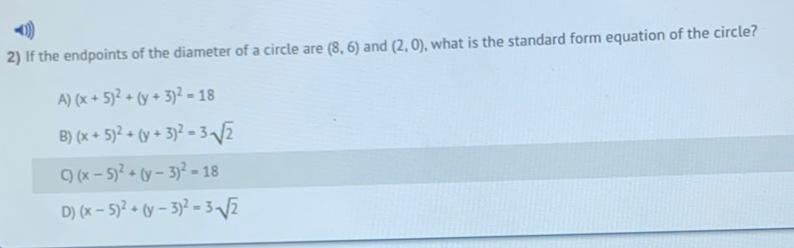Question:

# If the endpoints of the diameter of a circle are (8,6) and (2.0), what is the standard form equation of the circle? A) (x + 5)^2 + (y + 3)^2 =18 B) (x + 5)^2 + (y + 3)^2 = 3√2 c)(x - 5)^2 + (y - 3)^2If the endpoints of the diameter of a circle are (8,6) and (2.0), what is the standard form equation of the circle? A) (x + 5)^2 + (y + 3)^2 =18 B) (x + 5)^2 + (y + 3)^2 = 3√2 c)(x - 5)^2 + (y - 3)^2 =18 D)(x - 5)^2 + (y - 3)^2 = 3√2